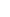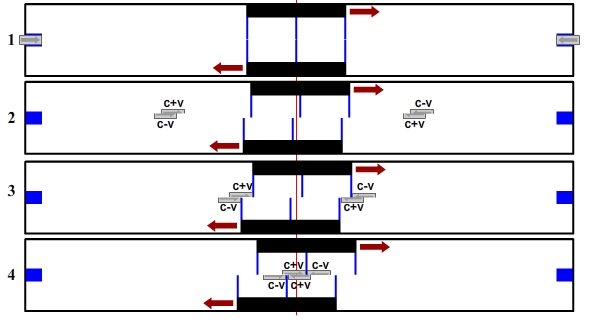37. PROOF OF (C+V) (C-V) MATHEMATICSAt the beginning of the book, we obtained (c+v) (c-v) mathematics logically based on the principles of physics. Then, starting from this mathematics unity with Doppler Shift, we discussed many topics in order. I think this much of evidence and their compatibility with each other should be sufficient. However, there is more. (c+v) (c-v) mathematics is strong enough to prove its own existence with a physics proof. In this chapter, we are going to obtain (c+v) (c-v) mathematics in a very tough way.The proof of (c+v) (c-v) mathematics has two stages. In the first stage, we will see that the speed of light is not c relative to all reference systems. In the second stage, (c+v) (c-v) mathematics will come before us forcefully.FIRST STAGE The speed of light is not c relative to all reference systems.Im going to prove it by using a realistic device as the one below.Lets get to know the device: There are two sleds moving at constant speed. Light sensors are placed in the middle points of the sleds. The sensors are sensitive to light coming from both sides. There are light sources on both ends of the device. The device works with the symmetry principle. The line that goes through the middle point of the device is the symmetry axis. The speed of the sleds and their distance to the symmetry axis always equal. Both lamps are lit simultaneously relative to the symmetry axis. Therefore, whatever happens on the left side of the symmetry axis happens on the right side in the exact same way.The question that leads to the proof is this: While the sleds are moving towards the symmetry axis when should be turned on the lights so that the lights reach the sensors in the middle of the sleds from both sides simultaneously?There is only one answer that The Electromagnetic Theory and The Theory of Relativity can give to this question. At the moment the sensors reach the symmetry axis, the lights that are sent from both sides should also reach the symmetry axis, as well. If the moment of emission of the lights is arranged in such a way, the condition for the lights to reach the sensors from both sides simultaneously will be met.We see the situation described in the figures below. In the first figure, the lights are sent while the sleds are moving towards the symmetry axis. In the second figure, the sensors and the lights met at the symmetry axis.It is not possible for The Electromagnetic Theory and The Theory of Relativity to find another answer to the question above. Thus, we have grabbed them from their weakest points. Now, our job is extremely simple.First, lets show that the answer they have given is wrong. Our first job is to place extra sensors to both sides of the sleds so that they are at the same distance to the sensor in the middle.Now lets evaluate the new situation with a short logical reasoning.The figure on the left below: Assume that we place a lamp on each side of the sled. When the lamps are turned on simultaneously relative to the sensor in the middle, the lights reach the sensor at the same time. This situation is independent of the speed of the sled that is in inert motion. The lights will reach the sensor simultaneously whether the sleds stand still or move. Is it correct? Yes. Is is possible to object to this? No. There can be objections, but then that wouldnt be physics. A person who calls himself a physicist should not object to this because, in case of an objection, there will be no such thing as The Electromagnetic Theory or The Theory of Relativity anymore.The figure on the right above: Now that we placed extra sensors to the sled, now we are facing such a situation: The lights that come towards the sled from both sides should first reach the sensors on the sides simultaneously. This situation is no different than placing lamps to both sides of the sled. The lights will reach the sensor simultaneously only and only if this condition is met.Now we are ready and we hit the point where they are the weakest by asking the same question again:  While the sleds are moving towards the symmetry axis, when should be turned on the lights so that the lights reach the sensors in the middle of the sleds from both sides simultaneously?The sensors we placed on the sides of the sled has now made The Electromagnetic Theory and The Theory of Relativity unable to answer this question.1, 2, 3, 4, 5, 6, 7, 8, 9 and 10! Knockout!They are knocked out because there is no option that they can suggest with the theoretical foundation that they have. We see why they ran out of options below:Since the sleds are in motion, if the lights reach the sensors on the sides simultaneously (Figure 1-a), they will not reach the sensors in the middle simultaneously (Figure 1-b). If the lights reach the sensors in the middle simultaneously (Figure 2-a), then they havent reached the sensors on the sides simultaneously before (Figure 2-b).Then what is the way to a solution? The first thing to say for this question is this: The Electromagnetic Theory and The Theory of Relativity do not have a way out of this. And there will be no solution unless the idea that the speed of light is constant relative to all reference systems is abandoned.SECOND STAGE Emergence of (c+v) (c-v) mathematics.Id like to direct your attention to the fact that it is not possible to argue that the lights cannot reach the sensors in the middle simultaneously in any way. It is obvious that such a situation should absolutely exist. On the other hand, we saw that the lights should reach the sensors on the sides of the sled simultaneously, as well. The way to the solution that can answer the question will lead us to the correct theory.And at this stage (c+v) (c-v) mathematics comes out and declares itself. It says: I am the way to the solution!. We see how (c+v) (c-v) mathematics easily achieves this below.1- When the middle sensors are on the symmetry axis, lights set out. 2- Electromagnetic waves that make up the light form (c+v) and (c-v) signal groups according to their arrival targets. 3- The lights reach the sensors on the sides of the sleds simultaneously.  4- The lights reach the middle sensors simultaneously.Where is the proof here?There is only one option for the moment when the lights are turned on. The lights should be turned on when the middle sensors of the sleds are on the symmetry axis. At this moment, the distances of the middle sensors to the light sources are equal. Relative to the middle sensors own reference systems, since the INCOMING signals that come to them from both sides are c, travel time will be equal as well and the lights will reach the middle sensors simultaneously. Since the distances of the sensors on the sides of the sled to the middle sensor are the same, the lights will reach these sensors simultaneously, as well. Speeds of the sleds, lengths of the sleds, the distance of the light sources have no effect on the result.At the moment when the lights are turned on, the sleds and the middle sensors are on the symmetry axis.  Travel time of the lights to the middle sensor = d0/c Travel time of the light to the sensors on the sides of the sled = (d0-d1)/c On the other hand, a light beam is a cluster that electromagnetic waves form together. Since each electromagnetic wave moves at c speed relative to their own arrival target, the OUTGOING lights that go towards the sleds that move in different directions at v speed are compulsory divided into two. Whichever sled the electromagnetic wave goes to, it will be in the group that goes to that sled. The speeds of these groups relative to the light sources are (c+v) if the sled is moving away and (c-v) if the sled is approaching. Here we see the (c+v) (c-v) mathematics emerge as an obligation.Emergence of (c+v) (c-v) mathematicsWhat led to the proof is that there is only one option for the moment when the lights are turned on. When the middle sensors of the sleds are on the symmetry axis, the lights should set out. Such a result is a situation that The Electromagnetic Theory and The Theory of Relativity can never suggest with the theoretical foundation that they have. According to these theories, at the moment the lights set out, the middle sensors should be in a situation in which they havent reached the symmetry axis yet. However, the sensors we placed on the sides of the sled left these theories, which can easily give answers when there is only one sensor in the middle of the sled, without a way out. And the proof is established on this strategy. First, leave them without a way out, then explain the solution. The solution can only be reached by (c+v) (c-v) mathematics that Alice Law has.All stages of this proof have been covered within the topics of the book. If you have come here in the natural flow of the book, you are not surprised in the topic of the solution by Alice Law.The original form of this proof is registered with the date October 23, 2000, and under no:31001 in the 37th Notary Office in Beyoglu, Istanbul, the Republic of Turkey. My studies on Alice Law started with this proof.